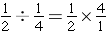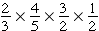# Number Properties

In earlier grades, you learned to use the order of operations to simplify numerical expressions. There are other ways to help you organize or reorder and regroup numbers, which make them easier to work with. These rules, which have been tested and proven over hundreds of years, are called number properties. They can be used alone or together to make simplifying numerical and algebraic expressions easier and these properties work for ALL numbers. We will be using a combination of the commutative, associative, identity, and distributive properties to help simplify expressions and solve problems.

First, we will look at a property called the Commutative Property. This property can be used with addition and multiplication and is all about order. Think of the trip to your mailbox and back. You can commute from your house to the mailbox and commute from the mailbox to your house. There is no difference in the scenery or the distance for either trip. You are simply changing your position.

The commutative property of addition states that changing the order of addends does not change the sum: a + b = b + a

The commutative property of multiplication states that changing the order of the factors does not change the product: a * b = b * a

### InvestigateKaren went to the county fair and was able to ride 4 rides, eat 3 treats, and watch 2 shows. She was able to get 9 things for her money. Using the commutative property to show how these things can be added, just switch the order.

4 + 3 + 2 = 9

3 + 4 + 2 = 9

2 + 4 + 3 = 9

### Investigate

Kristi practiced the piano 2 times each day for 30 minutes each practice period. She practiced 3 days per week for 4 weeks. One expression for the total number of minutes Kristi practiced the piano is: 2 * 30 * 3 * 4

Can this expression be rewritten another way using the commutative property? Will the total number of practice hours change if the commutative property is used?

Now, let us take a look at a property called the Associative Property. This property can also be used only with addition and multiplication and is all about grouping. This property does not change the order of any number, however, it allows for the use of parenthesis to group numbers together. This property is especially helpful when simplifying algebraic expressions because it allows you to group the like terms together for combinations. Think of your association with friends in school and outside of school. Sometimes, you change groups depending on your surroundings.

The associative property of addition states that for all real numbers, the sum is the same, regardless of the grouping:

(a + b) + c = a + (b +c)

The associative property of multiplication states that for all real numbers, the product is the same, regardless of the grouping:

a x (b x c) = (a x b) x c

Investigate

4 + (10 + 8) = 4 + 18 = 22

(4+ 10)+ 8 = (14) + 8 =22

In this case, the placement of the parenthesis has changed, but the solution remains the same.

### InvestigateJoy went on a rafting trip with her youth group. She saw that the cost was going to be \$10 per person, in addition to \$5 for the bus and an additional \$2 for a bottle of water. One expression for the total amount this trip will cost Joy is: (10 + 5) + 2 = \$17

Can this expression be rewritten another way using the associative property? Will the total cost of the trip change if the associative is used?

## What’s next?

There are a couple of other properties that help you write equivalent expressions. These are known as identity properties. First, you can use the Identity Property of Zero, also known as the Identity Property of Addition, by adding 0 to any number or variable. When you do this, the sum is the same as the original number. Additionally, you can use the Identity Property of One, also known as the Identity Property of Multiplication, by multiplying any number or variable by 1. Once again, the product will have the same identity as the original number. These properties are often combined with other number properties to simplify expressions.

Inverse strategies will be helpful in simplifying expressions throughout this course. Inverse simply reverses a set or operation. When you work with signed numbers, you can see that the inverse of  X is – X and vice versa. When you add the inverse to a number, your sum will always be 0. This is called the additive inverse property.

Dividing by a number is the same as multiplying by its reciprocal, also known as its inverse:Can you see why this works?. This method is called the multiplicative inverse property.

### Investigate

The following expression shows how the additive inverse property can be used to simplify.

3[2 + (-2)] the value of this expression is 0.

The following expression is an example of how the multiplicative inverse property can be used to simplify.is a great example of how we can use our properties to simplify an expression quickly. First, do you see anything in this expression that might be an inverse fraction? We can see thatis equal to, which simplifies to 1.

Now we can use the identity property of one to remind us that the other 2 fractions in the problem will keep their identity.

Therefore,is the same as.

All that is left for us to do is to multiply these two fractions to get our product.

Finally, we can use the distributive property to express sums and products by breaking apart one of the numbers and writing it as a sum or difference. You might associate this with the delivery of a newsletter being distributed to several places. You distribute a number or variable to several other numbers or variables to simplify an expression.

You can watch videos or read more about these properties at Khan Academy if they aren’t clear to you. You can use the menu on the left to find the different properties.

Here is a chart that might help you learn these properties and how they can be used individually or together to simplify both numerical and algebraic expressions.

For any real numbers a, b, and c.## Practice – Name the Property

1. When two numbers are multiplied together, the product is the
same regardless of the order of the multiplicands. For example a x b
= b x a.
2. When three or more numbers are added, the sum is the same
regardless of the grouping of the addends. For example (a + b) + c
= a + (b + c)
3. Adding 0 to any number leaves it unchanged. For example a + 0
= a.
4. When three or more numbers are multiplied, the product is the
same regardless of the grouping of the multiplicands. For example
(a x b) x c = a x (b x c)
5. The sum of two numbers times a third number is equal to the
sum of each addend times the third number. For example a x (b +
c) = a x b + a x c
6. The product of any number and one is the original number. For
example a x 1 = a.
7. When two numbers are added, the sum is the same regardless of
the order of the addends. For example a + b = b + a
8. The multiplicative inverse of a number, a is 1/a ; so that a x 1/a
= 1.

(source)